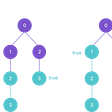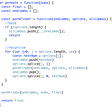# Algorithm Problem Review #4: Multiple Pointers

Another week and yet another algorithm problem from the Colt Steele Udemy course. This week I was working on multiple pointers, a technique I can use to solve problems without having to doubly loop. Instead, I would create pointers that would correspond to an index or a position and then move that pointer in any direction I need based on a condition.

# Multiple Pointers — countUniqueValues

Implement a function called countUniqueValues, which accepts a sorted array, and counts the unique values in the array. There can be negative numbers in the arry, but it will always be sorted

## Example

Inputs: [1, 1, 1, 2]
Output: 2

Inputs: [1, 2, 2, 3, 4, 4, 5, 11 , 12, 13]
Output: 8

Inputs: []
Output: 0

Inputs: [-2, -1, -1, 0, 1]
Output: 4

`function countUniqueValues(arr){}`

# Understanding The Problem

Before starting to code, I restated the problem: I need to create a function that accepts a sorted array from the smallest number to the largest number. I’ll name that arr. Then I have to count all the unique values in the array and return that total at the end. If arr is empty, then the total number of unique values is 0.

# Breaking it Down

Commenting on my function the things I need to do for this problem.

`function countUniqueValues(arr){  // Do something...  // Return a number of total unique values}`

“Do something”: The important part to remember for this problem is that the numbers in arr are sorted from low to high. So my plan here is to look at the first two numbers in the array and place a pointer i and j variable on both of them, respectively. It should look something like this:

`// so i will start in the 0 index of arr i[1,1,1,3,3,5]   j// And j will start on the 1st index of arr`

My plan here is to compare arr[j] to arr[i]. If arr[j] and arr[i] are the same then I will move j up to the next index until they aren’t the same value:

`// i is STILL at the beginning of arr and hasn't moved yet i[1,1,1,3,3,5]       j// j is now at an element that doesn't match with i's element`

When arr[i] and arr[j] don’t match, we will move i up one index and change arr[i] to be the same value as arr[j]:

`// i is moved to the next index and changed the value here to arr[j]   i[1,3,1,3,3,5]       j`

Then we can continue and move j to the next index in arr and continue checking if they match or don’t match. When j hits the end of the array we should have:

`// i is over the 2nd index of arr     i[1,3,5,3,3,5]           j// j is at the last index of arr`

The elements from arr to arr[i] are all the unique numbers from low to high, we can ignore the extra elements after arr[i]. We can count how many unique numbers we have by adding 1 to the i variable which is at the index of the largest number in our sorted array.

So now, to put that in JavaScript terms here’s what we’ll do:

`function countUniqueValues(arr){// To automatically return 0 if the array is empty  if(arr.length === 0){    return 0  }// i and j are the indices that the pointers will start at  let i = 0;  let j = 1;// moving the pointer variables through the array (see above)  while(j < arr.length){    if(arr[i] === arr[j]){      j++    } else if (arr[i] !== arr[j]){      i++      arr[i] = arr[j]      j++    }  }// return a number of total unique values which is index i + 1  return i + 1}`

# SOLVED — with O(n) time complexity

`function countUniqueValues(arr){  if(arr.length === 0){    return 0  }  let i = 0;  let j = 1;  while(j < arr.length){    if(arr[i] === arr[j]){      j++    } else if (arr[i] !== arr[j]){      i++      arr[i] = arr[j]      j++    }  }  return i + 1}`

# My Thoughts + Refactoring

This problem was tricky for me to work with. I’ll definitely need more practice with multiple-pointers problems. After watching Colt Steele solve this problem I found a big way I could’ve refactored to avoid repeating myself, especially with the j++ part. I could use a for loop instead of a while loop so it could add 1 to j instead of me explicitly writing it after the if-else statements and making my j variable.

`function countUniqueValues(arr){  if(arr.length === 0){    return 0  }  let i = 0;  for(let j = 1; j < arr.length; j++){    if(arr[i] !== arr[j]){      i++      arr[i] = arr[j]    }  }  return i + 1}`

# Happy Coding!

--

--

--

## More from Ajak Cyer

Software Engineering Student at Flatiron NYC. Learning day by day 💻

Love podcasts or audiobooks? Learn on the go with our new app.

## Upload to Azure Blob Storage with Angular 8## Mock Functions — not People## Set Up Your React Native App to Offer In-App Purchases and Subscriptions## Stripe Checkout Integration with Angular## Create A Hash Anchor Link Effect With React-Router## Ajak Cyer

Software Engineering Student at Flatiron NYC. Learning day by day 💻

## Array Stepper Algorithm Problem## Algorithms: Recursion/BackTracking, Permutations## Linked List in Javascript (Data Structures)